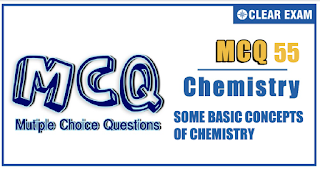## [LATEST]\$type=sticky\$show=home\$rm=0\$va=0\$count=4\$va=0

IIT JEE exam which consists of JEE Main and JEE Advanced is one of the most important entrance exams for engineering aspirants. The exam is held for candidates who are aspiring to pursue a career in the field of engineering and technical studies. Chemistry is important because everything you do is chemistry! Even your body is made of chemicals. Chemical reactions occur when you breathe, eat, or just sit there reading. All matter is made of chemicals, so the importance of chemistry is that it's the study of everything..

Q1.10 mL of a gaseous hydrocarbon is exploded with 100 mL of oxygen. The residual gas on cooling is found to measure 95 mL of which 20 mL is absorbed by caustic soda and the remainder by alkaline pyrogallol. The formula of the hydrocarbon is
•  CH4
•  C2H6
•  C2H4
•  C2H2
Solution
(d) C_x H_y+ (x+y/4) O_2→xCO_2+y/2 H_2 O 1 mL (x+y/4)mL x mL y/2 ml 10 mL 10(x+y/4)mL 10 x mL Volume absorbed by KOH = Volume of CO_2 Volume of CO_2=20 mL ∴10 x=20 x = 2 mL=2 mol Volume obsorbed by alkaline pyrogallol = volume of O_2 = 95-20 = 75 mL Excess of O_2=75 mL Total O_2=100 mL Volume of O_2reacted =100-75=25 mL ∴10(x+y/4)=25 ml ∴y=2 Formula of hydrocarbon =C_2 H_2

•  A
•  B
•  C
•  D
Solution

(b) PbCrO_4+HCl⟶PbCl_2+H_2 CrO_4 2CrO_4^(2-)+2H^+⟶Cr_2 O_7^(2-)+H_2 O orange

•  A
•  B
•  C
•  D
•  1X10-3
•  6.02X10^20
•  22.4X10^-3
•  6.02X3X10^2
Solution
(b) 0.018 mL=0.018 g (density of water=1g/mL) =0.018/18=0.001 mol =6.02×10^20 molecules

Q5.Per cent yield of NH_3 in the following reaction is 80% NH_2 CONH_2+2NaOH □(→┴( ∆ ) ) Na_2 CO_3+2NH_3 6 g NH_2 CONH_2 reacts with 8 g NaOH to form NH_3
•  3.4 g
•  2.72 g
•  4.25 g
•  11.2 g
Solution
(b) NH_2 CONH_2+2NaOH→2NH_3 60g 80 g 2×17=34 g 6 g 8 g 3.4 g If yield is 100% NH_3 formed=3.4 g If yield is 80% NH_3 formed=3.4×80/100=2.72 g

Q6. The weight of MnO_2 and the volume of HCl of specific gravity 1.2 g mL^(-1) and 4% nature by weight, needed to produce 1.78 L of Cl_2 at STP. The reaction involved is: MnO_4+4HCl→MnCl_2+2H_2 O+Cl_2
•  0.48 L
•  0.24 L
•  0.12 L
•  0.06 L
Solution
(b) MnO_2+4HCl→MnCl_2+2H_2 O+Cl_2 Moles 0.08 4×0.8 1.78/22.4=0.08 =0.32 ⇒ W_(MnO_2 )=0.08×87 g =6.96 g ((10×4×1.2)/36.5)×V_L=0.32 ⇒V_L=0.24 L

Q7.0.1 g of metal combines with 46.6 mL of oxygen at STP. The equivalent weight of metal is
•  12
•  24
•  6
•  36
Solution
(a) 1 mol of O_2 = 4 eq. of O 22400 mL of O_2 = 4 eq. of O 46.6 mL of O_2=4/22400×46.6 = 0.00832 eq. Equivalent of metal = Equivalent of O Weight/Ew=0.00832 0.1/E=0.00832 ∴E=0.1/0.00832=12.0

Q8.3.4 g sample H_2 O_2 solution containing x % H_2 O_2by weight requires xmL of a KMnO_4 solution for complete oxidation under acidic condition. The normality of KMnO_4 solution is
•  1N
•  2N
•  3N
•  0.5N
Solution
(b) 100 g H_2 O_2 sample solution contains x g of H_2 O_2 3.4 g of solution contains =x/100×3.4 Weight of H_2 O_2=(3.4 x)/100 Eq ofH_2 O_2=(3.4 x)/100×1/17 mEq of H_2 O_2=(3.4 x)/(100×17)×1000=34x/17=2x mEq of KMnO_4=x×N ∴x×N=2x N=2

Q9.A spherical ball of radius 7 cm contains 56% iron. If density is 1.4 g/cm^3, number of moles of Fe present approximately is
•  10
•  15
•  20
•  25
Solution
(c) Mass/Volume=Density ∴Mass of spherical ball=V×d =4/3 πr^3×d =4/3×22/7×(7)^3×1.4 =2012.27 g pure Fe content(56%)=2012.27×56/100 g=1126.87 g Thus, moles of Fe =20.12

Q10.Mass of one atom of an element is 6.64×10^(-23) g. This is equal to
•  6.64×10^(-23) u
•  40.0u
•  1/40u
•  6.64 u
Solution
(b) Mass of one atom =6.64×10^(-23) g Thus, atomic mass =6.64×10^(-23)×6.02×10^23=40#### Written by: AUTHORNAME

AUTHORDESCRIPTION## Want to know more

Please fill in the details below:

## Latest NEET Articles\$type=three\$c=3\$author=hide\$comment=hide\$rm=hide\$date=hide\$snippet=hide

Name

ltr
item
BEST NEET COACHING CENTER | BEST IIT JEE COACHING INSTITUTE | BEST NEET & IIT JEE COACHING: SOME BASIC CONCEPTS OF CHEMISTRY Quiz-55
SOME BASIC CONCEPTS OF CHEMISTRY Quiz-55
https://1.bp.blogspot.com/-BojUhqHTfrU/YOkx_n0oXGI/AAAAAAAAVlc/KiE0Y7H72o0_c-Vbxdpvr55rQ3vYy7WpwCLcBGAsYHQ/s320/Screenshot%2B2021-07-10%2Bat%2B10.43.52%2BAM.png
https://1.bp.blogspot.com/-BojUhqHTfrU/YOkx_n0oXGI/AAAAAAAAVlc/KiE0Y7H72o0_c-Vbxdpvr55rQ3vYy7WpwCLcBGAsYHQ/s72-c/Screenshot%2B2021-07-10%2Bat%2B10.43.52%2BAM.png
BEST NEET COACHING CENTER | BEST IIT JEE COACHING INSTITUTE | BEST NEET & IIT JEE COACHING
https://www.cleariitmedical.com/2021/07/some-basic-concepts-of-chemistry-quiz-55.html
https://www.cleariitmedical.com/
https://www.cleariitmedical.com/
https://www.cleariitmedical.com/2021/07/some-basic-concepts-of-chemistry-quiz-55.html
true
7783647550433378923
UTF-8

STAY CONNECTED